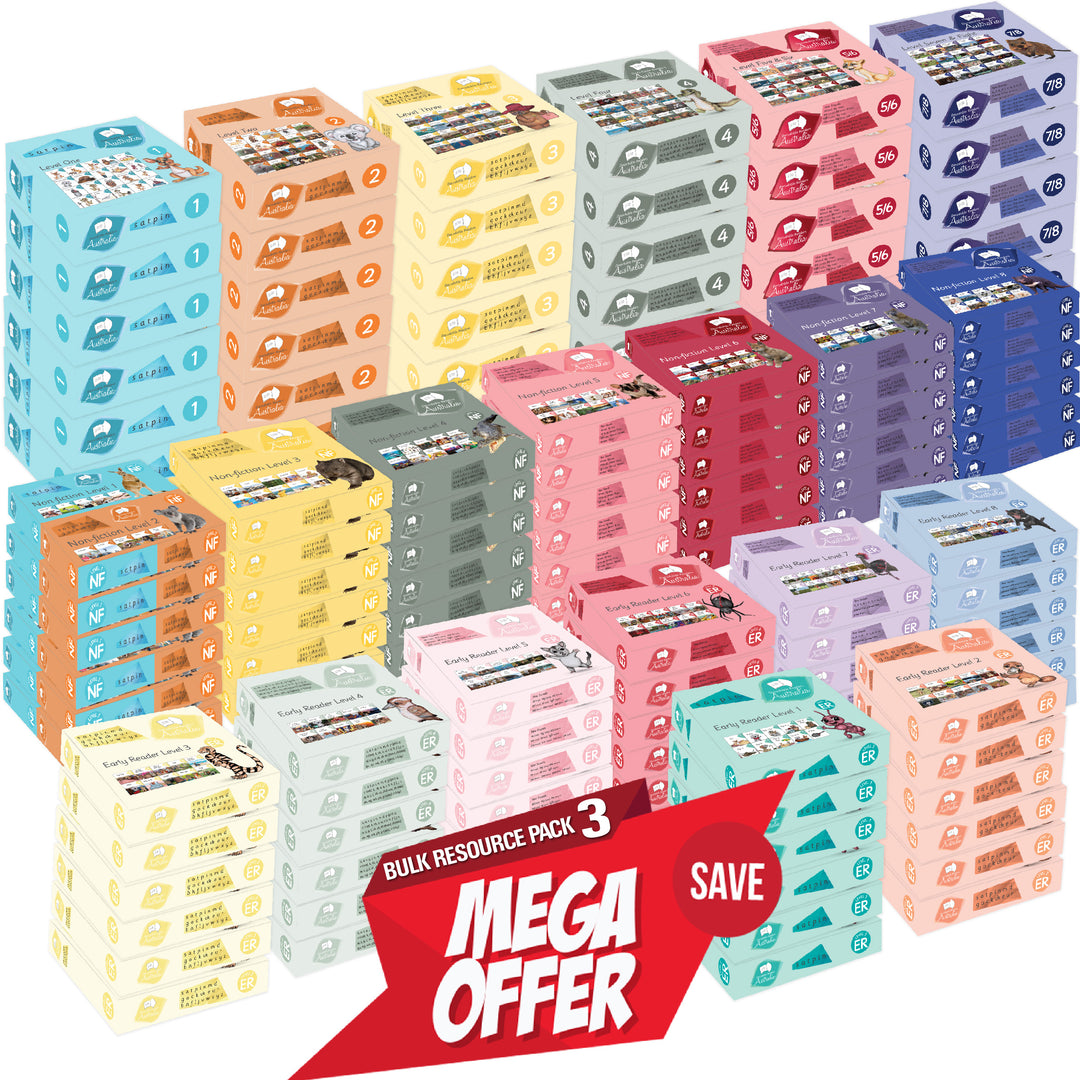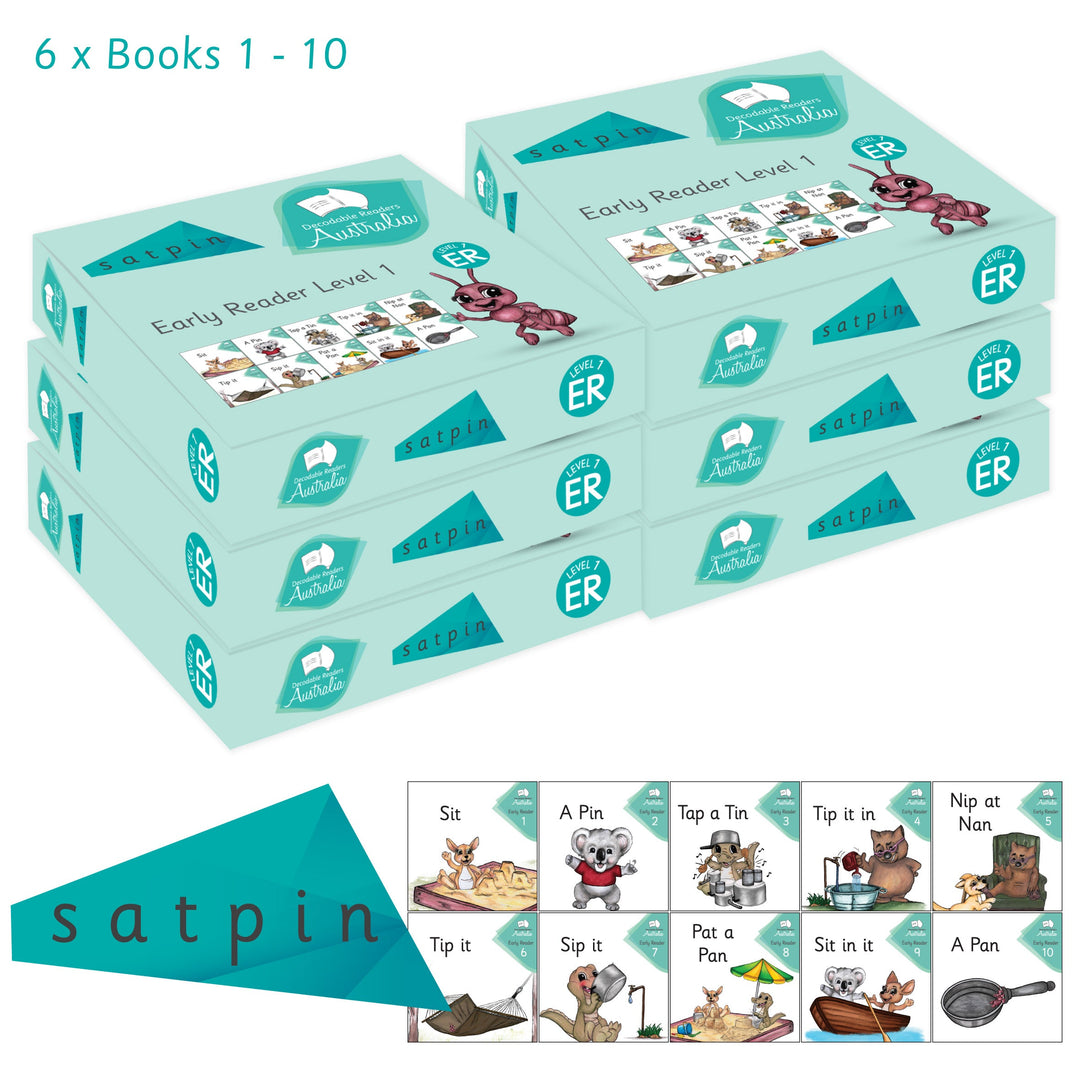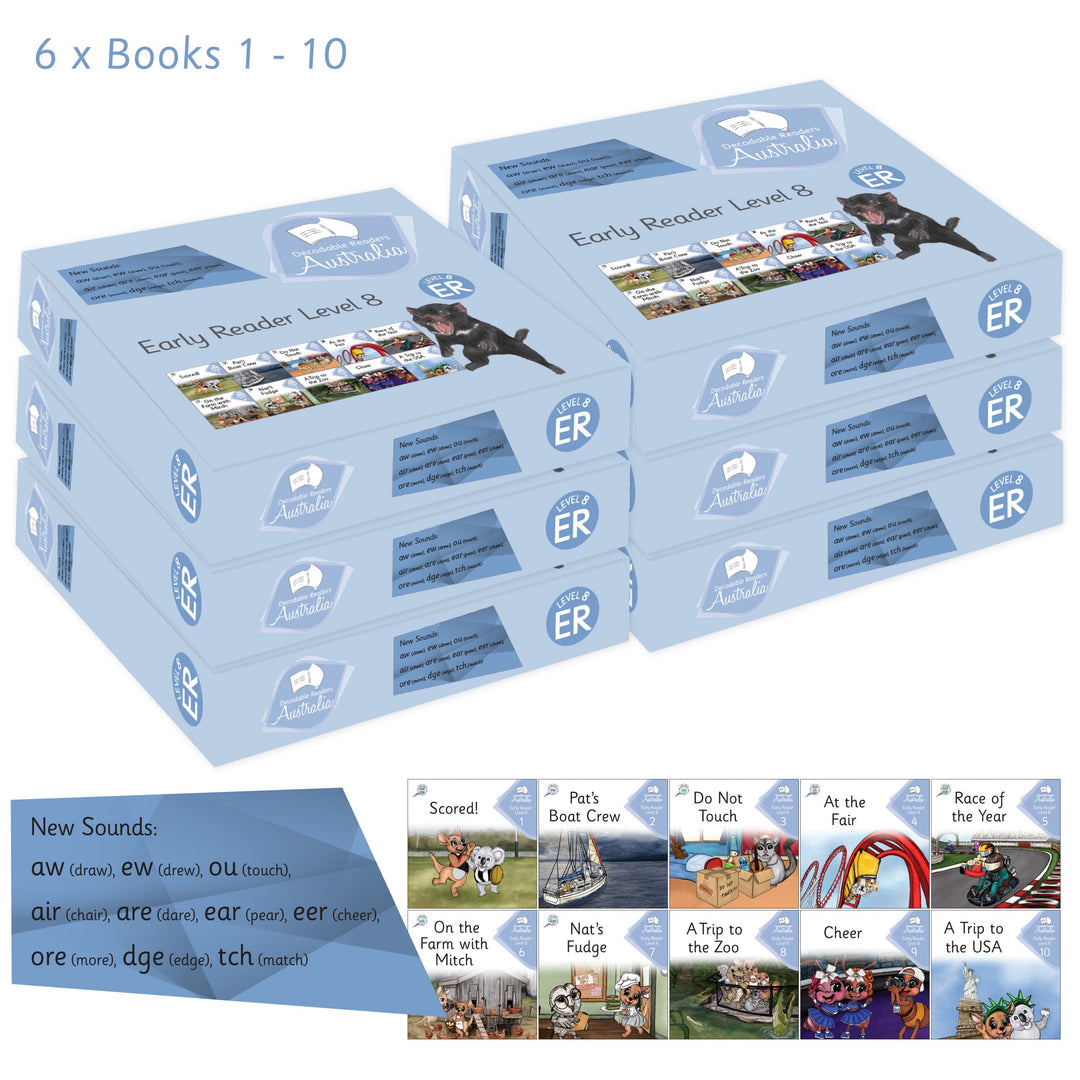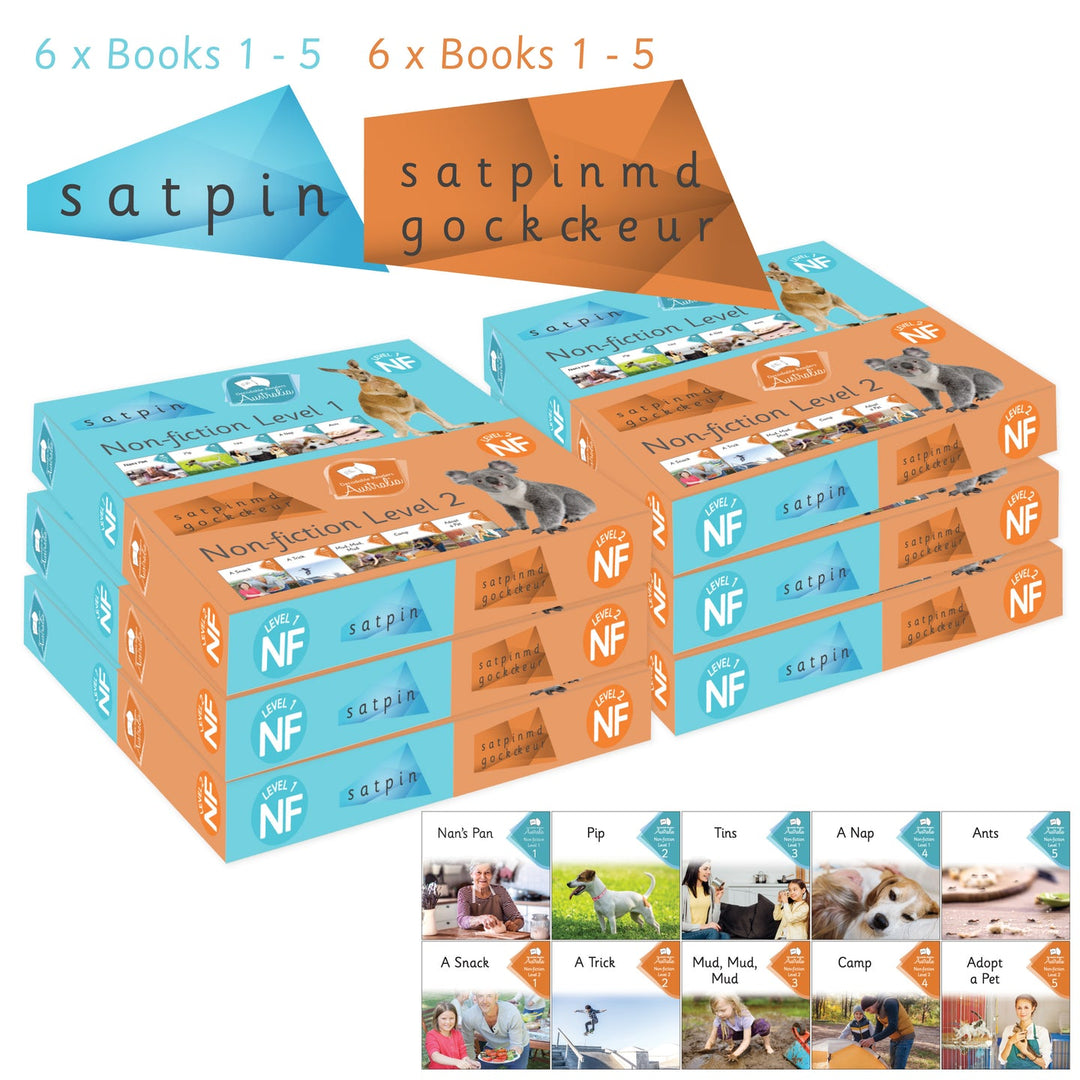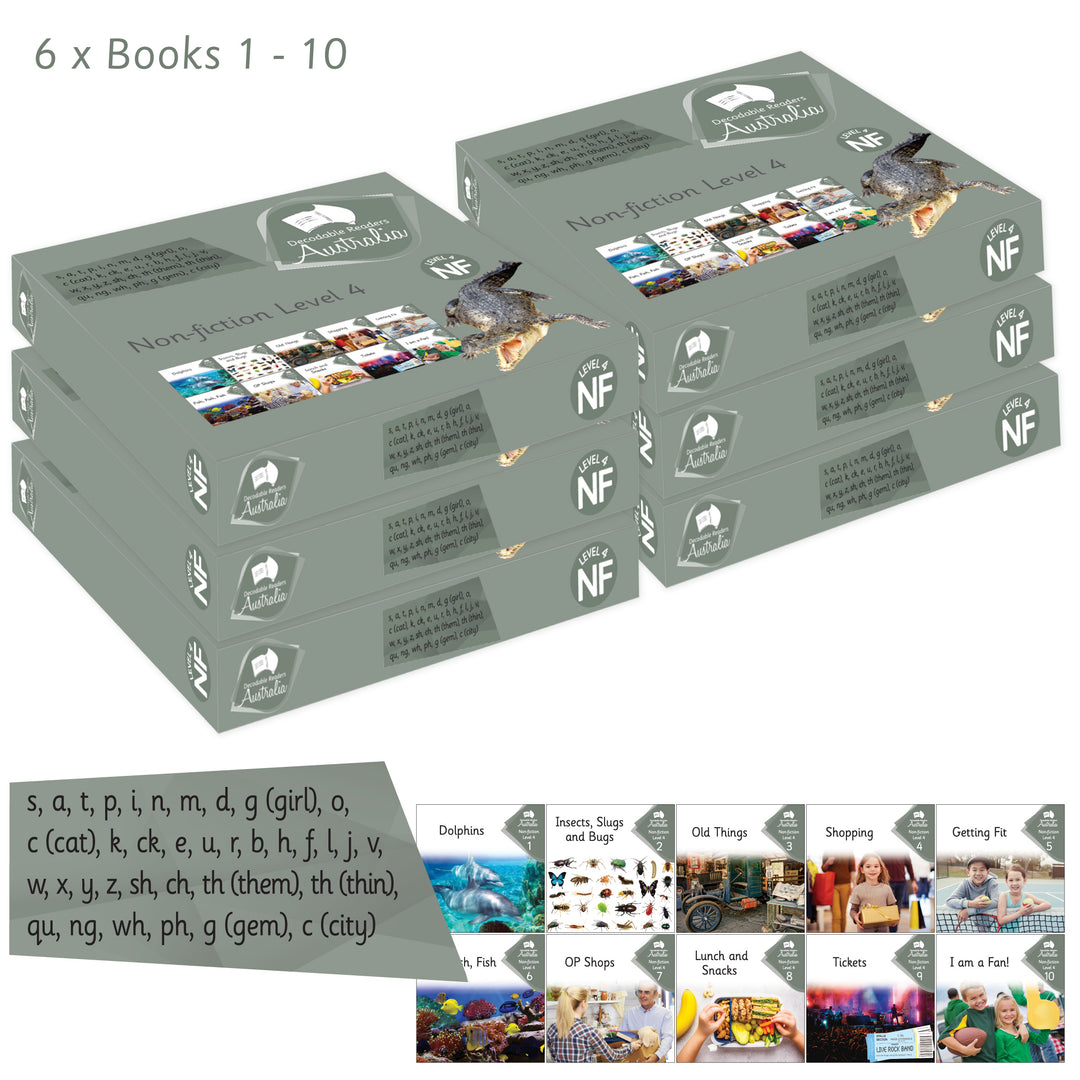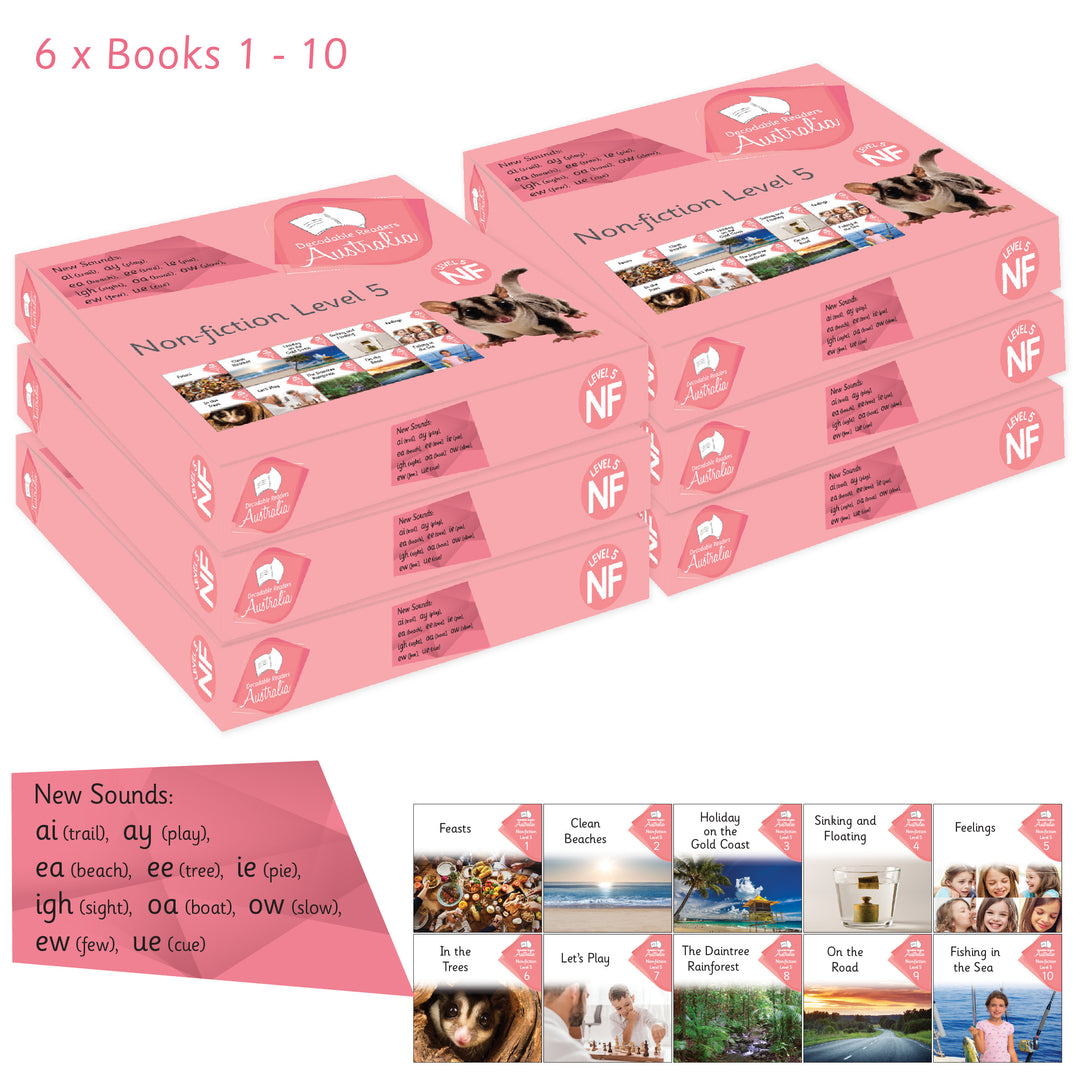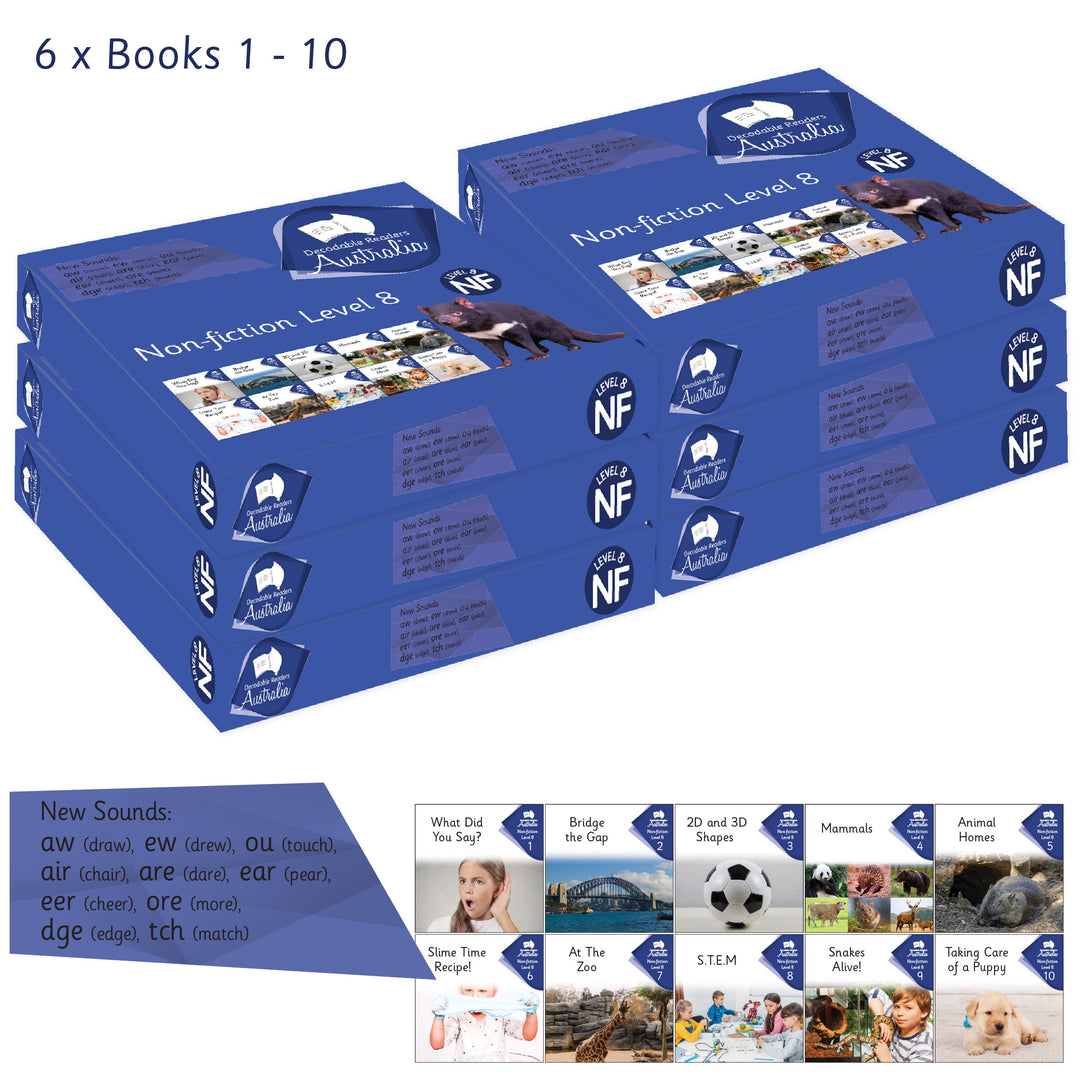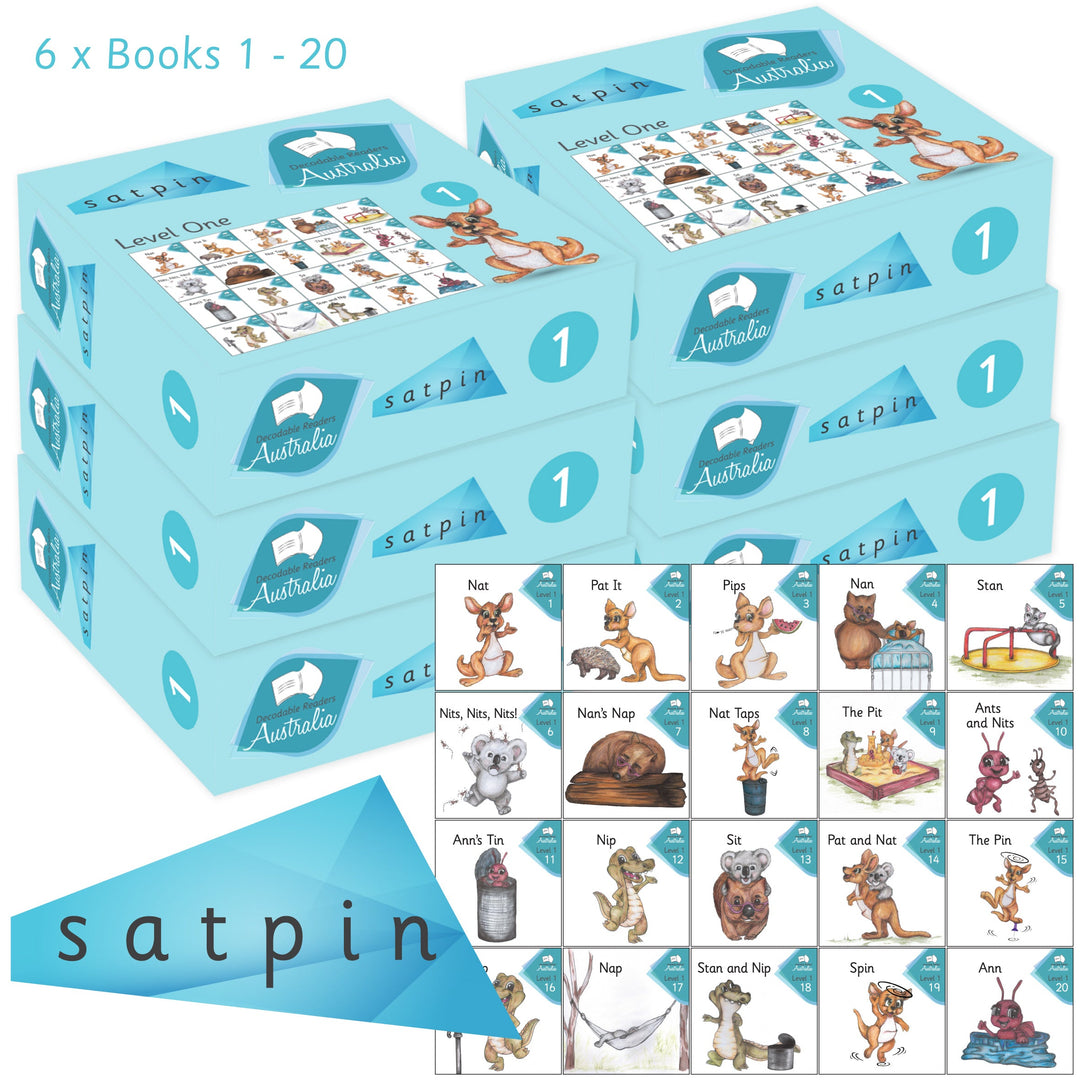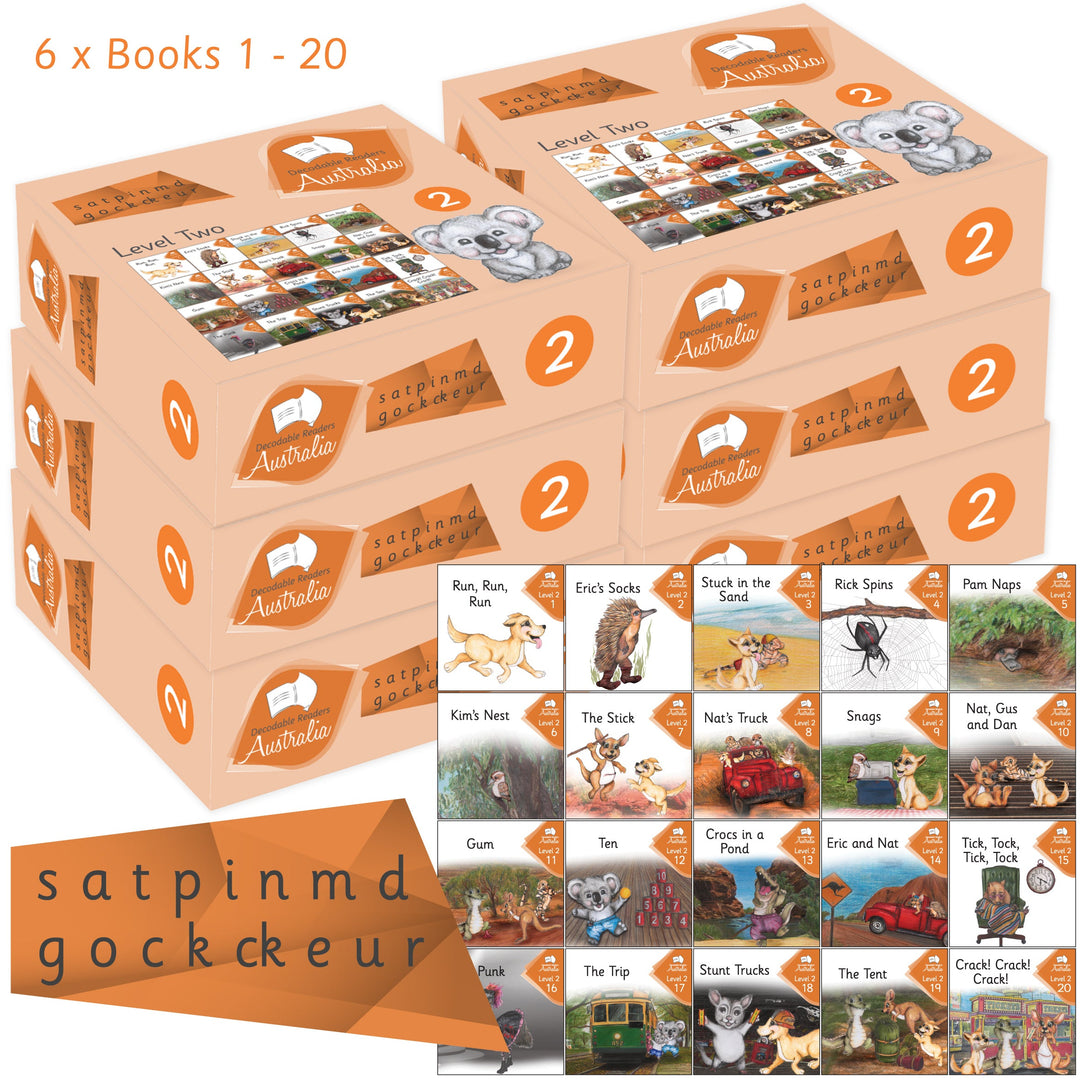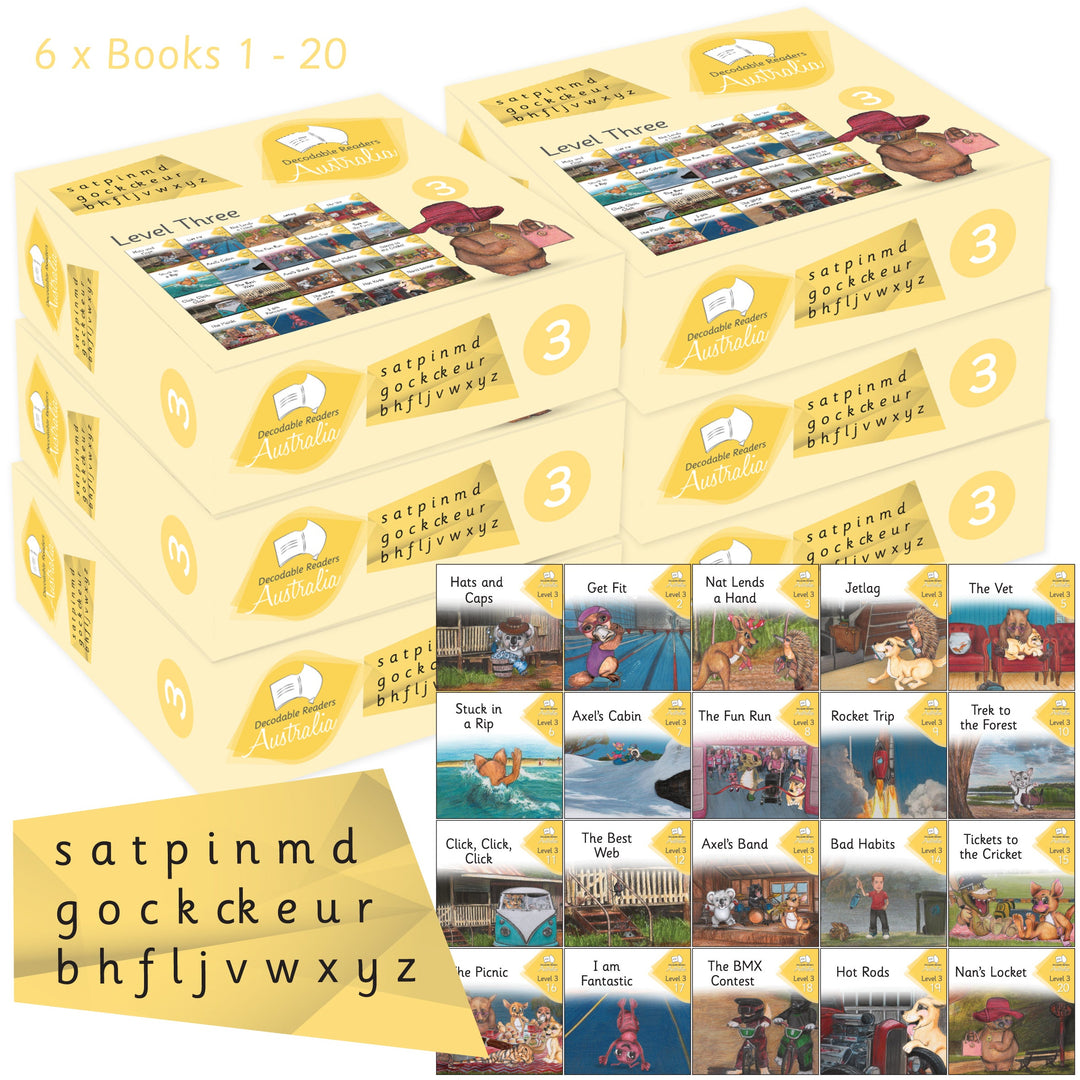# Bulk Resource Pack 3 - PRE ORDER: DELIVERED SEPTEMBER\$7,995.00 AUD

\$9,315.00 AUD

Bulk Resource Pack 3
Levels 1 to 8 (Early Readers + Nonfiction + Main Series) 6 copies of each title.

PRE ORDER: DELIVERED SEPTEMBER

Resource your Early Years Classrooms, Learning Support and Library!

6 x Complete Individual Early Readers Sets =
6x Level 1 = 60 Books
6x Level 2 = 60 Books
6x Level 3 = 60 Books
6x Level 4 = 60 Books
6x Level 5 = 60 Books
6x Level 6 = 60 Books
6x Level 7 = 60 Books
6x Level 8 = 60 Books

= 480 Books

6 x Complete Individual Nonfiction Readers Sets =
6x Level 1/2 = 60 Books
6x Level 3 = 60 Books
6x Level 4 = 60 Books
6x Level 5 = 60 Books
6x Level 6 = 60 Books
6x Level 7 = 60 Books
6x Level 8 = 60 Books

= 420 Books

6 x Complete Individual Main Series Set =
6x Level 1 = 120 Books
6x Level 2 = 120 Books
6x Level 3 = 120 Books
6x Level 4 = 120 Books
6x Level 5/6 = 120 Books
6x Level 7/8 = 120 Books
= 720 Books